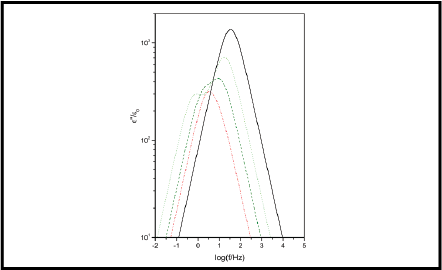# Electrical impedance of an electrolytic cell in the presence of generation and recombination of ions### Abstract

The electrical impedance of an insulating solid containing ions is evaluated in the presence of the generation and recombination of ions. The case of perfectly blocking electrodes is considered. It is shown that in the case where the diffusion coefficients of the positive and negative ions are equal, the expression for the electrical impedance coincides with the one valid in the absence of the generation and recombination of ions, with the bulk density of ions fixed by the chemical reaction describing the generation and recombination of ions. In particular, in the low frequency region, the resistance tends to the value corresponding to the plateau, and the reactance diverges as the inverse of the frequency. The case where the neutral particles, that by decomposition generate the ions, and the positive ions have a vanishing small diffusion coefficient with respect to that of the negative ions is also considered. In this situation, in the series representation, the resistance of the cell presents two plateaus. One in the low frequency region is related to the generation-recombination phenomenon. The other, with a resistance smaller than the previous one, ends at the frequency of Debye. This result is of some importance because it could be the mechanism able to explain the experimental data obtained with hydrogels doped with KCl.

Type